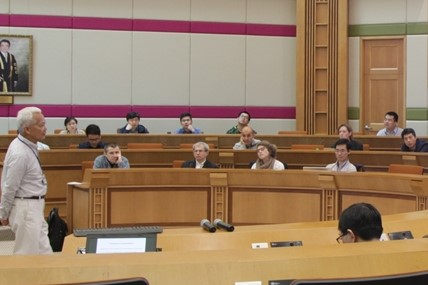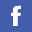## Colloquium/Seminar

 Year Month 2023 Jan     Feb 2022 Jan     Feb     Mar     Apr     May     Jun     Jul     Aug     Oct     Nov     Dec 2021 Feb     Mar     Apr     May     Jun     Jul     Aug     Sep     Oct     Nov     Dec 2020 Jan     May     Jun     Jul     Aug     Sep     Oct     Nov     Dec 2019 Jan     Feb     Mar     Apr     May     Jun     Jul     Aug     Oct     Nov

### Event(s) on January 2023

• Tuesday, 10th January, 2023

 Title: Ordinary Kriging with Variable Range Parameter Speaker: Mr LIANG Yi, Department of Mathematics, Hong Kong Baptist University, Hong Kong Time/Place: 14:30  -  16:30 Zoom, Meeting ID: 973 9604 7470 Passcode: 801036 Abstract: Spatial data interpolation is a general problem with many applications. In statistics, there is an interpolation technique, kriging, also known as the best linear unbiased prediction. It assumes that the surface of spatial data is a realization from a random field. The best predictor of kriging is the mean conditioned on the observation data. When the Gaussian process assumption is added, the best predictor will be a linear form, which is corresponding to the best linear predictor. It is much simplified to solve a linear problem. However, the variogram modeling problem which is the central task in kriging prediction is still tricky, where the parameter selection problem is a big issue. In this thesis, we investigate a variable scheme for parameter selection of variogram modeling in order to improve the accuracy of the point estimation of ordinary kriging. The forms of several well-known variogram models such as Gaussian, exponential, and spherical all have a similar structure. We say they belong to a range parameter family, where we can define the proposed variable range parameter. The way of constructing the variable parameter is inspired by that varying the distances between points of the region yields the same result as varying the range parameter. The strategy keeps the variogram model symmetric and conditionally negative definite, which is important in model interpretation. The variable parameter method is more flexible than the traditional constant parameter approach. Numerical experiments show that the proposed variable method outperforms the classical constant method.

• Tuesday, 31st January, 2023

 Title: Optimal regression learning: minimax rate of convergence, sparsity, and model compression Speaker: Professor Yuhong Yang, School of Statistics, University of Minnesota Time/Place: 10:30  -  11:30 Zoom, Meeting ID: 956 5475 3160 Abstract: Minimax-rate optimality plays a foundational role in theory of statistical and machine leaning. In the context of regression, some important questions are: i) What determines the minimax-rate of convergence for regression estimation? ii) Is it possible to construct estimators that are simultaneously minimax optimal for a countable list of function classes? iii) In high-dimensional linear regression, how does different kinds of sparsity affect the rate of convergence? iv) How do we know if a pre-trained deep neural network model is compressible? If so, by how much? In this talk, we will address the above questions. After reviewing on the determination of minimax rate of convergence, we will present on minimax optimal adaptive estimation for high-dimensional regression learning under both hard and soft sparsity setups, taking advantage of recent sharp sparse linear approximation bounds. An application on model compression in neural network learning will be given.

• Tuesday, 31st January, 2023

 Title: Multiple Change Point Detection in Tensors Speaker: Jiaqi Huang, School of Statistics, Beijing Normal University Time/Place: 16:30  -  17:30 FSC1217, Meeting ID: 966 9643 8487 Abstract: We propose two novel criteria for detecting change structures in tensor data. To measure the difference between any two adjacent tensors and to handle both dense and sparse model structures of the tensors, we define a signal-screening Frobenius distance for the moving sums of tensor data and a mode-based signal-screening Frobenius distance for the moving sums of slices of tensor data. The latter is particularly useful when some mode is not suitable to be included in the former distance. Based on these two sequences, we construct two signal statistics using the ratios with adaptive-to-change ridge functions. The estimated number of changes and their estimated locations are consistent to the corresponding true quantities in certain senses. The results hold when the size of the tensor and the number of change points diverge at certain rates, respectively. Numerical studies are conducted to examine the finite sample performances of the proposed methods. We also analyze two real data examples for illustration.

All years### Conferences and Workshops

We organize conferences and workshops every year. Hope we can see you in future.### Lecture Series

Details### Employment